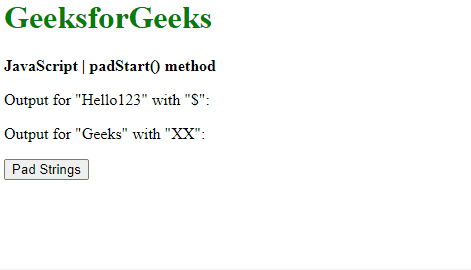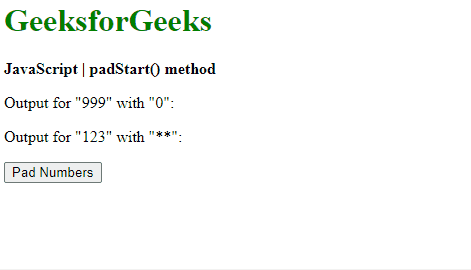Open in App
Not now

• Last Updated : 26 Dec, 2022

The padStart() method in JavaScript is used to pad a string with another string until it reaches the given length. The padding is applied from the left end of the string.

Syntax:

`string.padStart(targetLength, padString)`

Parameters: This method accepts two parameters as mentioned above and described below:

• targetLength: It is the length of the final string once the original string has been padded. If the value is less than the original string length, then the original string is returned.
• padString: It is the string that is to be padded with the original string. If this value is too long to be within the targetLength, it is truncated from the end. The default used string is the space character (” “).

Return Value: It returns the final string that is padded with the given string to the given length.

Example 1: This example uses the padStart() method to pad strings into another string.

## html

 `<``h1` `style``=``"color: green"``>``    ``GeeksforGeeks```` ` `<``b``>JavaScript | padStart() method`` ` `<``p``>Output for "Hello123" with "\$":``    ``<``span` `class``=``"output"``>```` ` `<``p``>Output for "Geeks" with "XX":``    ``<``span` `class``=``"output_2"``>```` ` `<``button` `onclick``=``"padStrings()"``>``    ``Pad Strings```` ` `<``script` `type``=``"text/javascript"``>``    ``function padStrings() {``        ``exString = "Hello123";``        ``exString2 = "Geeks";``     ` `        ``prepended_out =``            ``exString.padStart(10, "\$");``        ``prepended_out2 =``            ``exString2.padStart(10, "XX");``     ` `        ``document.querySelector('.output').textContent``                    ``= prepended_out;``         ` `        ``document.querySelector('.output_2').textContent``                    ``= prepended_out2;``    ``}```

Output:Example 2: This example uses the padStart() method to pad numbers into another number.

## html

 `<``h1` `style``=``"color: green"``>``    ``GeeksforGeeks```` ` `<``b``>JavaScript | padStart() method`` ` `<``p``>Output for "999" with "0":``    ``<``span` `class``=``"output"``>```` ` `<``p``>Output for "123" with "**":``    ``<``span` `class``=``"output_2"``>```` ` `<``button` `onclick``=``"padNumbers()"``>``    ``Pad Numbers```` ` `<``script` `type``=``"text/javascript"``>``    ``function padNumbers() {``        ``exNumber = 999;``        ``exNumber2 = 123;``     ` `        ``prepended_out``            ``= String(exNumber).padStart(5, "0");``         ` `        ``prepended_out2``            ``= String(exNumber2).padStart(5, "**");``     ` `        ``document.querySelector('.output').textContent``            ``= prepended_out;``         ` `        ``document.querySelector('.output_2').textContent``            ``= prepended_out2;``    ``}```

Output:We have a complete list of Javascript Strings, to check those please go through the Javascript String Complete reference article.

Supported Browsers: The browser supported by the padStart() method are listed below: